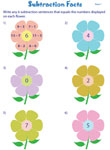# Subtraction Facts Worksheets

Build a strong foundation in subtraction with this vast collection of printable worksheets, exclusively drafted for kindergarten, 1st grade, and 2nd grade children. This series of worksheets contains subtraction facts from 0 through 9 in both vertical and horizontal formats. The difference between the numbers does not exceed 20. Make use of a heady mix of fun-filled theme-based subtraction facts, mixed subtraction facts and teacher templates displayed here. A few free worksheets are also up for grabs!

## Single Number Facts

Single Number Subtraction Facts: Column

This array of column subtraction worksheets contains simple subtraction facts from 0 to 9. Answers generated will not exceed 20.Fact 0:

Fact 1:

Fact 2:

Fact 3:

Fact 4:

Fact 5:

Fact 6:

Fact 7:

Fact 8:

Fact 9:

Single Number Subtraction Facts: Horizontal

This set of subtraction facts pdf worksheets contains problems positioned in the horizontal format.Fact 0:

Fact 1:

Fact 2:

Fact 3:

Fact 4:

Fact 5:

Fact 6:

Fact 7:

Fact 8:

Fact 9:

Subtraction Facts: Oyster Pearl Theme

This set of theme-based worksheets makes learning subtraction facts fun! Get kindergarten and grade 1 children to come up with five subtraction sentences to match the number (difference) displayed in the pearl.Subtraction Facts: Windmill Theme

Engage kids in learning subtraction facts with this series of printable worksheets! Kids should write five subtraction sentences on the blades to match the number (difference) that appears at the center of the windmill.## Mixed Number Facts

Mixed Subtraction Facts: Column

The numbers to be subtracted in this series of worksheets range from 0 to 9. The pdf worksheets are split into a combination of different range of numbers positioned in the vertical format.Fact 0 to 2:

Fact 0 to 3:

Fact 0 to 4:

Fact 0 to 5:

Fact 0 to 6:

Fact 0 to 7:

Fact 0 to 8:

Fact 0 to 9:

Mixed Subtraction Facts: Horizontal

A blend of subtraction facts from 0 to 9 is featured in this group of printable worksheets that are presented in the horizontal form. Verify the answer key to check your responses.Fact 0 to 2:

Fact 0 to 3:

Fact 0 to 4:

Fact 0 to 5:

Fact 0 to 6:

Fact 0 to 7:

Fact 0 to 8:

Fact 0 to 9:

Subtraction Facts: Flower Power Theme

Young learners of grade 1 and grade 2 should write six subtraction sentences to match the number (difference) displayed at the center of the flower. Teacher templates in color and monochrome are up for grabs!Color:

Printer-friendly:

Related Worksheets